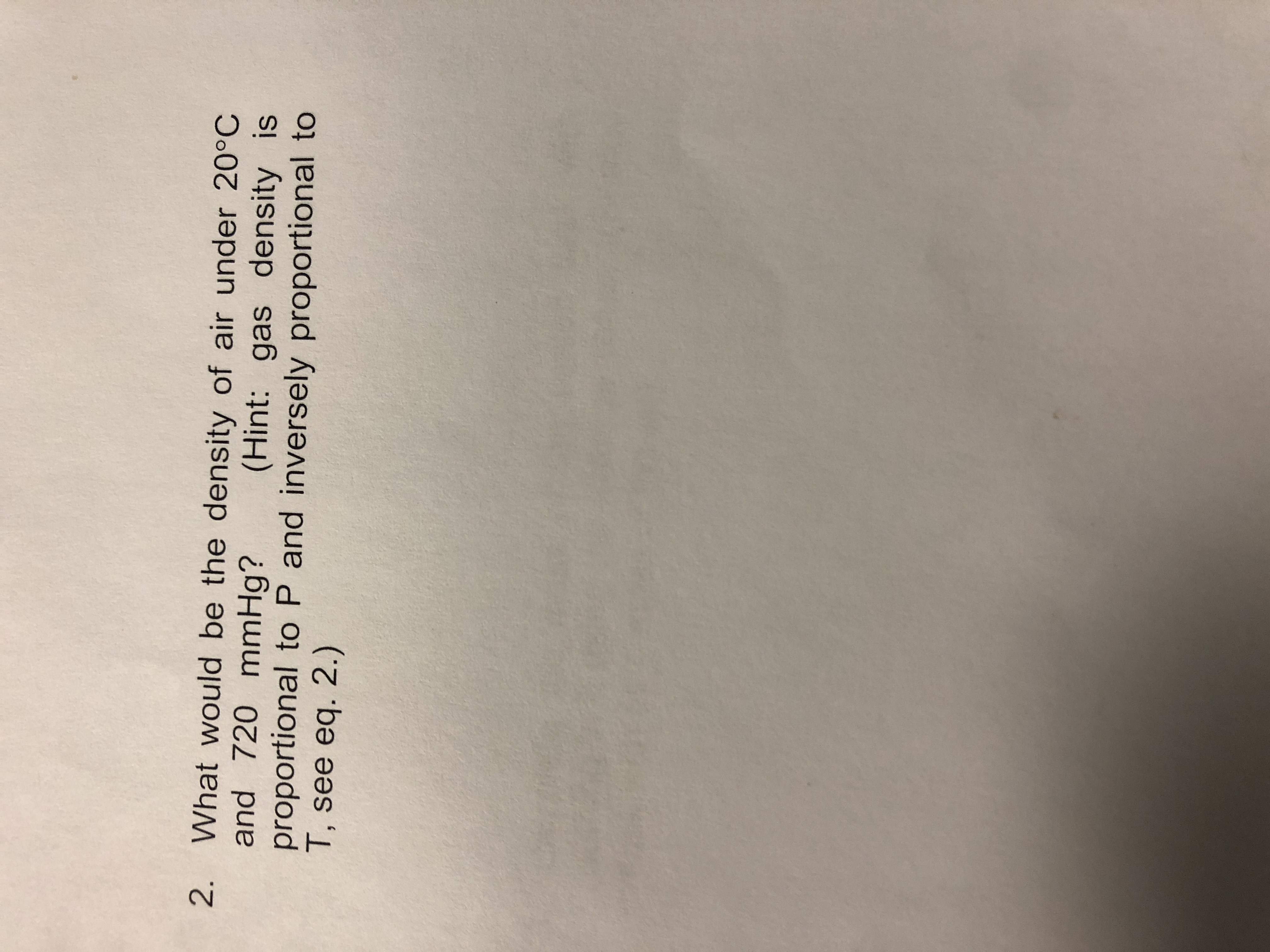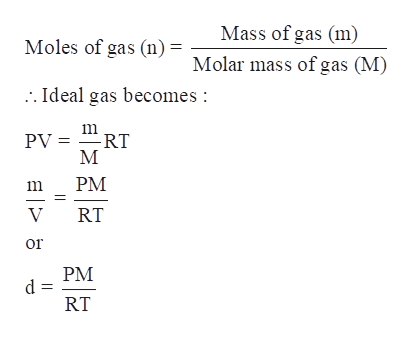# 2. What would be the density of air under 20°Cand 720 mmHg?proportional to P and inversely proportional toT, see eq. 2.)(Hint: gas density is

Question
1 viewshelp_outlineImage Transcriptionclose2. What would be the density of air under 20°C and 720 mmHg? proportional to P and inversely proportional to T, see eq. 2.) (Hint: gas density is fullscreen
check_circle

Step 1

The density of air under 20° C and 720 mmHg is to be calculated.

Step 2

Density of a substance is defined as the mass of substance divided by its volume.

Step 3

According to an ideal gas law:

PV = nRT

Where

P = Pressure of the gas

V = Volume occupied by gas

R...help_outlineImage TranscriptioncloseMass of gas (m) Moles of gas (n)- Molar mass of gas (M) .. Ideal gas becomes : m PV = RT M PM RT or PM RT E|> fullscreen

### Want to see the full answer?

See Solution

#### Want to see this answer and more?

Solutions are written by subject experts who are available 24/7. Questions are typically answered within 1 hour.*

See Solution
*Response times may vary by subject and question.
Tagged in

### Gas laws